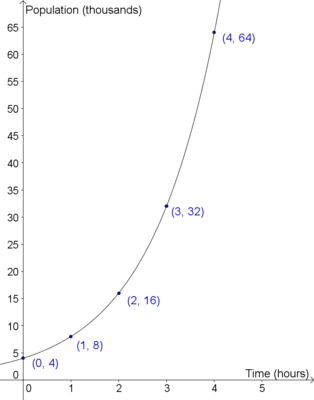# Extending the Definitions of Exponents, Variation 2

Alignments to Content Standards: F-LE.A N-RN.A.1

A biology student is studying bacterial growth. She was surprised to find that the population of the bacteria doubled every hour.

1. Complete the following table and plot the data.
 Hours into study Population (thousands) 0 1 2 3 4 4
2. Write an equation for $P$, the population of the bacteria, as a function of time, $t$, and verify that it produces correct populations for $t$ = 1, 2, 3, and 4.
3. The student conducting the study wants to create a table with more entries; specifically, she wants to fill in the population at each half hour. However, she forgot to make these measurements so she wants to estimate the values.

Instead she notes that the population increases by the same factor each hour, and reasons that this property should hold over each half-hour interval as well. Fill in the part of the table below that you've already computed, and decide what constant factor she should multiply the population by each half hour in order to produce consistent results. Use this multiplier to complete the table:

 Hours into study Population (thousands) 0 $\frac12$ 1 $\frac32$ 2 $\frac52$ 3 4
4. What if the student wanted to estimate the population every 20 minutes instead of every 30 minutes. What multiplier would be necessary to be consistent with the population doubling every hour? Use this multiplier to complete the following table:
 Hours into study Population (thousands) 0 $\frac13$ $\frac23$ 1 $\frac43$ $\frac53$ 2 4
5. Use the population context to explain why it makes sense that we define $2^{\frac{1}{2}}$ to be $\sqrt{2}$ and $2^{\frac{1}{3}}$ as $\sqrt{2}$.
6. Another student working on the bacteria population problem makes the following claim:
If the population doubles in 1 hour, then half that growth occurs in the first half-hour and the other half occurs in the second half-hour. So for example, we can find the population at $t=\frac{1}{2}$ by finding the average of the populations at $t=0$ and $t=1$.

Comment on this idea. How does it compare to the multipliers generated in the previous problems? For what kind of function would this reasoning work?

## IM Commentary

The goal of this task is to develop an understanding of why rational exponents are defined as they are (N-RN.1), however it also raises important issues about distinguishing between linear and exponential behavior (F-LE.1c) and it requires students to create an equation to model a context (A-CED.2)

## Solution

1.  Hours into study Population (thousands) 0 1 2 3 4 4 8 16 32 64

Students would be expected to find these values by repeatedly multiplying by 2. The plot below consists of the exponential function $P(t)=4\cdot 2^t$ which students will derive in the next part. The plot of the data alone would consist of the 5 plotted blue points.2. One possible equation is $P(t)=4\cdot 2^t$, since as we tallied above via repeated multiplication, we have $4\cdot 2^1=8$, $4\cdot 2^2=16$, $4\cdot 2^3=32$, $4\cdot 2^4=64$, etc.  Others include $P(t)=2^{t+2}$, $P(t)=2\cdot 2^{t+1}$, etc.

3.  Hours into study Population (thousands) 0 $\frac12$ 1 $\frac32$ 2 $\frac52$ 3 4 5.657 8 11.314 16 22.627 32

Let $x$ be the multiplier for the half-hour time interval. Then since going forward a full hour in time has the effect of multiplying the population by $x^2$, we must have $x^2=2$, and so the student needs to multiply by $\sqrt{2}$ every half hour.

4.  Hours into study Population (thousands) 0 $\frac13$ $\frac23$ 1 $\frac43$ $\frac53$ 2 4 5.010 6.350 8 10.079 12.699 16

Similarly, since waiting three 20-minute intervals should double the population, the new multiplier has to satisfy $x\cdot x\cdot x=2$, which gives $x^3=2$. So you would need to multiply by $\sqrt{2}$ every 20 minutes to have the effect of doubling every hour.

5. We already know that the equation for population is $P=4(2)^t$ when $t$ is a natural number. Given this, it's reasonable to use the expression $P(\frac{1}{2})=4(2)^{\frac{1}{2}}$ to define $2^{\frac{1}{2}}.$ However, we reasoned above that $P(\frac{1}{2})=4\cdot\sqrt{2}$, and equating the two gives $2^{\frac{1}{2}}=\sqrt{2}$. Similarly, equating the expression $P(\frac{1}{3})=2^{1/3}$ with the calculation $P(\frac{1}{3})=\sqrt{2}$ gives the reasonable definition $2^{1/3}=\sqrt{2}$.

6. The reasoning mistakenly assumes linear growth within each hour, i.e., that the amount of population growth is the same each half hour. We know instead that the percentage growth is constant, not the raw change in population. If we were to apply the faulty reasoning to the first hour, we would get the following values:

 Hours into study Population (thousands) 0 $\frac12$ 1 4 6 8

However, this does not have constant percentage growth: from $t = 0$ to $t = \frac12$ this population grew by 50% ($\text{ratio} = 1.5$), but then from $t = \frac{1}{2}$ to $t = 1$ the ratio is only $1.33$. If you graphed this data, instead of seeing a smoothly increasing curve, you would see a series of connected line segments of increasing slopes.